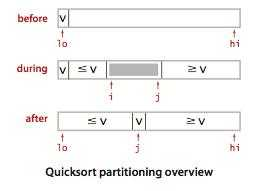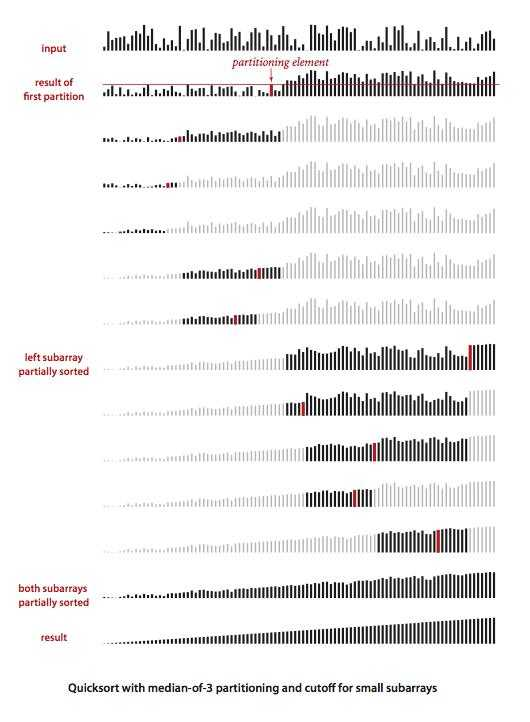# 快速排序

#### 切分

1. 一般策略是先随意地选取 a[lo] 作为切分元素，即那个会被排定的元素，然后我们
2. 从数组的左端开始向右扫描直到找到一个大于等于它的元素，
3. 再从数组的右端开始向左扫描直到找到一个小于等于它的元素。
4. 交换它们的位置
5. 递归1-4，当两个指针相遇时，我们就可以保证左指针 i 的左侧元素都不大于切分元素，右指针 j 的右侧元素都不小于切分元素。
6. 我们只需要将切分元素 a[lo] 和左子数组最右侧的元素（a[j]）交换然后返回 j 即可。
注：此时a[i]找到大于a[lo]的元素,a[j]找到小于a[lo]的元素，所以a[lo]只能和a[j]交换``````public static int partition(Comparable[] a, int lo, int hi) {
int i = lo, j = hi + 1;
Comparable v = a[lo];
while (true) {
while (less(a[++i], v)) {//找一个 比V大的数的index  i
if (i == hi) {
break;
}
}
while (less(v, a[--j])) {//找一个比v小的数的index   j
if (j == lo) {
break;
}

}
if (i >= j) {//如果i的 index 在j 的右边就不交换
break;
}
exch(a, i, j);
}
exch(a, lo, j);
return j;
}``````

#### 最好情况

``````public class Quick {
public static void sort(Comparable[] a) {
StdRandom.shuffle(a);   // 消除对输入的依赖
sort(a, 0, a.length - 1);
}

private static void sort(Comparable[] a, int lo, int hi) {
if (hi <= lo) return;
int j = partition(a, lo, hi); // 切分
sort(a, lo, j-1);             // 左半部分排序
sort(a, j+1, hi);             // 右半部分排序
}
}``````#### 特点

(0)
(0)